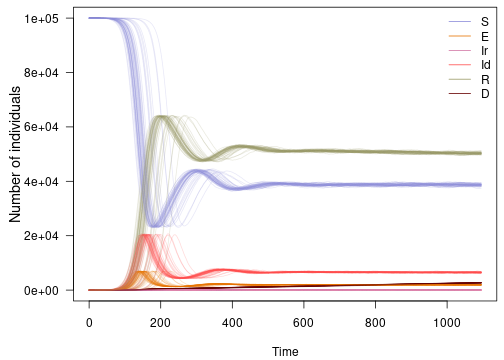# Discrete compartmental models in a nutshell

## From continuous to discrete time

In its simplest form, a SIR model is typically written in continuous time as:

$\frac{dS}{dt} = - \beta \frac{S_t I_t}{N_t}$

$\frac{dI}{dt} = \beta \frac{S_t I_t}{N_t} - \gamma I_t$

$\frac{dR}{dt} = \gamma I_t$

Where $$\beta$$ is an infection rate and $$\gamma$$ a removal rate, assuming 'R' stands for 'recovered', which can mean recovery or death.

For discrete time equivalent, we take a small time step $$t$$ (typically a day), and write the changes of individuals in each compartment as:

$S_{t+1} = S_t - \beta \frac{S_t I_t}{N_t}$

$I_{t+1} = I_t + \beta \frac{S_t I_t}{N_t} - \gamma I_t$

$R_{t+1} = R_t + \gamma I_t$

## Stochastic processes

The discrete model above remains deterministic: for given values of the rates $$\beta$$ and $$\gamma$$, dynamics will be fixed. It is fairly straightforward to convert this discrete model into a stochastic one: one merely needs to uses appropriate probability distributions to model the transfer of individuals across compartments. There are at least 3 types of such distributions which will be useful to consider.

### Binomial distribution

This distribution will be used to determine numbers of individuals leaving a given compartment. While we may be tempted to use a Poisson distribution with the rates specified in the equations above, this could lead to over-shooting, i.e. more individuals leaving a compartment than there actually are. To avoid infecting more people than there are susceptibles, we use a binomial distribution, with one draw for each individual in the compartment of interest. The workflow will be:

1. determine a per-capita probability of leaving the compartment, based on the original rates specified in the equations; if the rate at which each individual leaves a compartment is $$\lambda$$, then the corresponding probability of this individual leaving the compartment in one time unit is $$p = 1 - e^{- \lambda}$$.

2. determine the number of individuals leaving the compartment using a Binomial distribution, with one draw per individual and a probability $$p$$

As an example, let us consider transition $$S \rightarrow I$$ in the SIR model. The overall rate at which this change happens is $$\beta \frac{S_t I_t}{N_t}$$. The corresponding per susceptible rate is $$\beta \frac{I_t}{N_t}$$. Therefore, the probability for an individual to move from S to I at time $$t$$ is $$p_{(S \rightarrow I), t} = 1 - e^{- \beta \frac{I_t}{N_t}}$$.

### Poisson distribution

Poisson distributions will be useful when individuals enter a compartment at a given rate, from 'the outside'. This could be birth or migration (for $$S$$), or introduction of infections from an external reservoir (for $$I$$), etc. The essential distinction with the previous process is that individuals are not leaving an existing compartment.

This case is simple to handle: one just needs to draw new individuals entering the compartment from a Poisson distribution with the rate directly coming from the equations.

For instance, let us now consider a variant of the SIR model where new infectious cases are imported at a constant rate $$\epsilon$$. The only change to the equation is for the infected compartment:

$I_{t+1} = I_t + \beta \frac{S_t I_t}{N_t} + \epsilon - \gamma I_t$

where:

• individuals move from $$S$$ to $$I$$ according to a Binomial distribution $$\mathcal{B}(S_t, 1 - e^{- \beta \frac{I_t}{N_t}})$$

• new infected individuals are imported according to a Poisson distribution $$\mathcal{P}(\epsilon)$$

• individual move from $$I$$ to $$R$$ according to a Binomial distribution $$\mathcal{B}(I_t, 1 - e^{- \gamma})$$

### Multinomial distribution

This distribution will be useful when individuals leaving a compartment are distributed over several compartments. The Multinomial distribution will be used to determine how many individuals end up in each compartment. Let us assume that individuals move from a compartment $$X$$ to compartments $$A$$, $$B$$, and $$C$$, at rates $$\lambda_A$$, $$\lambda_B$$ and $$\lambda_C$$. The workflow to handle these transitions will be:

1. determine the total number of individuals leaving $$X$$; this is done by summing the rates ($$\lambda = \lambda_A + \lambda_B + \lambda_C$$) to compute the per capita probability of leaving $$X$$ $$(p_(X \rightarrow …) = 1 - e^{- \lambda})$$, and drawing the number of individuals leaving $$X$$ ($$n_{_(X \rightarrow …)}$$) from a binomial distribution $$n_{(X \rightarrow …)} \sim B(X, p_(X \rightarrow …))$$

2. compute relative probabilities of moving to the different compartments (using $$i$$ as a placeholder for $$A$$, $$B$$, $$C$$): $$p_i = \frac{\lambda_i}{\sum_i \lambda_i}$$

3. determine the numbers of individuals moving to $$A$$, $$B$$ and $$C$$ using a Multinomial distribution: $$n_{(X \rightarrow A, B, C)} \sim \mathcal{M}(n_{(X \rightarrow …)}, p_A, p_B, p_C)$$

# Implementation using odin

## Deterministic SIR model

We start by loading the odin code for a discrete, stochastic SIR model:

path_sir_model <- system.file("examples/discrete_deterministic_sir.R", package = "odin")

## Core equations for transitions between compartments:
update(S) <- S - beta * S * I / N
update(I) <- I + beta * S * I / N - gamma * I
update(R) <- R + gamma * I

## Total population size (odin will recompute this at each timestep:
## automatically)
N <- S + I + R

## Initial states:
initial(S) <- S_ini # will be user-defined
initial(I) <- I_ini # will be user-defined
initial(R) <- 0

## User defined parameters - default in parentheses:
S_ini <- user(1000)
I_ini <- user(1)
beta <- user(0.2)
gamma <- user(0.1)


As said in the previous vignette, remember this looks and parses like R code, but is not actually R code. Copy-pasting this in a R session will trigger errors.

We then use odin to compile this model:

sir_generator <- odin::odin(path_sir_model)
sir_generator

## function (I_ini = NULL, S_ini = NULL, beta = NULL, gamma = NULL,
##     user = list(I_ini = I_ini, S_ini = S_ini, beta = beta, gamma = gamma),
##     unused_user_action = NULL)
## <an 'odin_generator' function>
##   use coef() to get information on user parameters


Note: this is the slow part (generation and then compilation of C code)! Which means for computer-intensive work, the number of times this is done should be minimized.

The returned object sir_generator is a function that will generate an instance of the model:

x <- sir_generator()
x

## <odin_model>
##   Public:
##     contents: function ()
##     initial: function (step)
##     initialize: function (user = NULL, unused_user_action = NULL)
##     ir: {"version":"1.0.8","config":{"base":"discrete_determinis ...
##     run: function (step, y = NULL, ..., use_names = TRUE, replicate = NULL)
##     set_user: function (..., user = list(...), unused_user_action = NULL)
##     transform_variables: function (y)
##     update: function (step, y)
##   Private:
##     core: list
##     discrete: TRUE
##     dll: discrete_deterministic_sir_0865cb82
##     init: 1000 1 0
##     interpolate_t: NULL
##     n_out: 0
##     name: discrete_deterministic_sir
##     output_order: NULL
##     ptr: externalptr
##     update_metadata: function ()
##     use_dde: NULL
##     user: I_ini S_ini beta gamma
##     variable_order: list
##     ynames: step S I R


x is an ode_model object which can be used to generate dynamics of a discrete-time, deterministic SIR model. This is achieved using the function x$run(), providing time steps as single argument, e.g.: sir_col <- c("#8c8cd9", "#cc0044", "#999966") x$run(0:10)

##       step         S        I         R
##  [1,]    0 1000.0000 1.000000 0.0000000
##  [2,]    1  999.8002 1.099800 0.1000000
##  [3,]    2  999.5805 1.209517 0.2099800
##  [4,]    3  999.3389 1.330125 0.3309317
##  [5,]    4  999.0734 1.462696 0.4639442
##  [6,]    5  998.7814 1.608403 0.6102138
##  [7,]    6  998.4604 1.768530 0.7710541
##  [8,]    7  998.1076 1.944486 0.9479071
##  [9,]    8  997.7198 2.137811 1.1423557
## [10,]    9  997.2937 2.350191 1.3561368
## [11,]   10  996.8254 2.583469 1.5911558

x_res <- x$run(0:200) par(mar = c(4.1, 5.1, 0.5, 0.5), las = 1) matplot(x_res[, 1], x_res[, -1], xlab = "Time", ylab = "Number of individuals", type = "l", col = sir_col, lty = 1) legend("topright", lwd = 1, col = sir_col, legend = c("S", "I", "R"), bty = "n")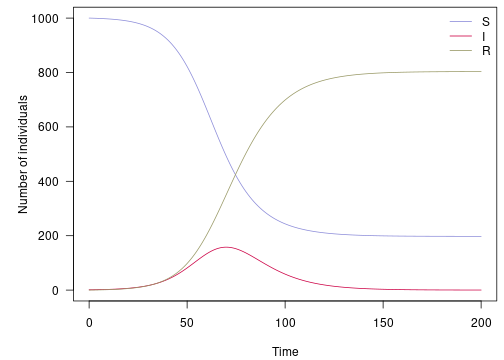## Stochastic SIR model The stochastic equivalent of the previous model can be formulated in odin as follows: path_sir_model_s <- system.file("examples/discrete_stochastic_sir.R", package = "odin")  ## Core equations for transitions between compartments: update(S) <- S - n_SI update(I) <- I + n_SI - n_IR update(R) <- R + n_IR ## Individual probabilities of transition: p_SI <- 1 - exp(-beta * I / N) # S to I p_IR <- 1 - exp(-gamma) # I to R ## Draws from binomial distributions for numbers changing between ## compartments: n_SI <- rbinom(S, p_SI) n_IR <- rbinom(I, p_IR) ## Total population size N <- S + I + R ## Initial states: initial(S) <- S_ini initial(I) <- I_ini initial(R) <- 0 ## User defined parameters - default in parentheses: S_ini <- user(1000) I_ini <- user(1) beta <- user(0.2) gamma <- user(0.1)  We can use the same workflow as before to run the model, using 10 initial infected individuals (I_ini = 10): sir_s_generator <- odin::odin(path_sir_model_s) sir_s_generator  ## function (I_ini = NULL, S_ini = NULL, beta = NULL, gamma = NULL, ## user = list(I_ini = I_ini, S_ini = S_ini, beta = beta, gamma = gamma), ## unused_user_action = NULL) ## <an 'odin_generator' function> ## use coef() to get information on user parameters  x <- sir_s_generator(I_ini = 10)  set.seed(1) x_res <- x$run(0:100)
par(mar = c(4.1, 5.1, 0.5, 0.5), las = 1)
matplot(x_res[, 1], x_res[, -1], xlab = "Time", ylab = "Number of individuals",
type = "l", col = sir_col, lty = 1)
legend("topright", lwd = 1, col = sir_col, legend = c("S", "I", "R"), bty = "n")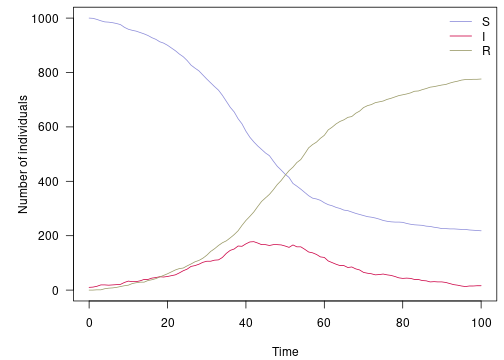This gives us a single stochastic realisation of the model, which is of limited interest. As an alternative, we can generate a large number of replicates using arrays for each compartment:

path_sir_model_s_a <- system.file("examples/discrete_stochastic_sir_arrays.R", package = "odin")

## Core equations for transitions between compartments:
update(S[]) <- S[i] - n_SI[i]
update(I[]) <- I[i] + n_SI[i] - n_IR[i]
update(R[]) <- R[i] + n_IR[i]

## Individual probabilities of transition:
p_SI[] <- 1 - exp(-beta * I[i] / N[i])
p_IR <- 1 - exp(-gamma)

## Draws from binomial distributions for numbers changing between
## compartments:
n_SI[] <- rbinom(S[i], p_SI[i])
n_IR[] <- rbinom(I[i], p_IR)

## Total population size
N[] <- S[i] + I[i] + R[i]

## Initial states:
initial(S[]) <- S_ini
initial(I[]) <- I_ini
initial(R[]) <- 0

## User defined parameters - default in parentheses:
S_ini <- user(1000)
I_ini <- user(1)
beta <- user(0.2)
gamma <- user(0.1)

## Number of replicates
nsim <- user(100)
dim(N) <- nsim
dim(S) <- nsim
dim(I) <- nsim
dim(R) <- nsim
dim(p_SI) <- nsim
dim(n_SI) <- nsim
dim(n_IR) <- nsim

sir_s_a_generator <- odin::odin(path_sir_model_s_a)
sir_s_a_generator

## function (I_ini = NULL, S_ini = NULL, beta = NULL, gamma = NULL,
##     nsim = NULL, user = list(I_ini = I_ini, S_ini = S_ini, beta = beta,
##         gamma = gamma, nsim = nsim), unused_user_action = NULL)
## <an 'odin_generator' function>
##   use coef() to get information on user parameters

x <- sir_s_a_generator()

set.seed(1)
sir_col_transp <- paste0(sir_col, "66")
x_res <- x$run(0:100) par(mar = c(4.1, 5.1, 0.5, 0.5), las = 1) matplot(x_res[, 1], x_res[, -1], xlab = "Time", ylab = "Number of individuals", type = "l", col = rep(sir_col_transp, each = 100), lty = 1) legend("left", lwd = 1, col = sir_col, legend = c("S", "I", "R"), bty = "n")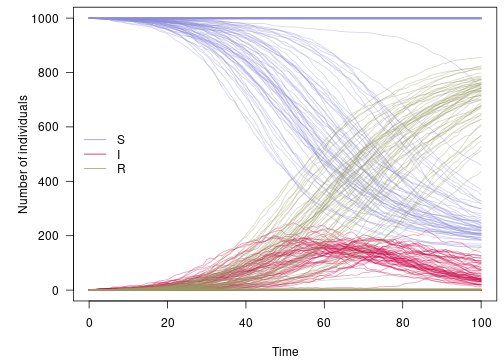## A stochastic SEIRDS model This model is a more complex version of the previous one, which we will use to illustrate the use of all distributions mentioned in the first part: Binomial, Poisson and Multinomial. The model is contains the following compartments: • $$S$$: susceptibles • $$E$$: exposed, i.e. infected but not yet contagious • $$I_R$$: infectious who will survive • $$I_D$$: infectious who will die • $$R$$: recovered • $$D$$: dead There are no birth of natural death processes in this model. Parameters are: • $$\beta$$: rate of infection • $$\delta$$: rate at which symptoms appear (i.e inverse of mean incubation period) • $$\gamma_R$$: recovery rate • $$\gamma_D$$: death rate • $$\mu$$: case fatality ratio (proportion of cases who die) • $$\epsilon$$: import rate of infected individuals (applies to $$E$$ and $$I$$) • $$\omega$$: rate waning immunity The model will be written as: $S_{t+1} = S_t - \beta \frac{S_t (I_{R,t} + I_{D,t})}{N_t} + \omega R_t$ $E_{t+1} = E_t + \beta \frac{S_t (I_{R,t} + I_{D,t})}{N_t} - \delta E_t + \epsilon$ $I_{R,t+1} = I_{R,t} + \delta (1 - \mu) E_t - \gamma_R I_{R,t} + \epsilon$ $I_{D,t+1} = I_{D,t} + \delta \mu E_t - \gamma_D I_{D,t} + \epsilon$ $R_{t+1} = R_t + \gamma_R I_{R,t} - \omega R_t$ $D_{t+1} = D_t + \gamma_D I_{D,t}$ The formulation of the model in odin is: path_seirds_model <- system.file("examples/discrete_stochastic_seirds.R", package = "odin")  ## Core equations for transitions between compartments: update(S) <- S - n_SE + n_RS update(E) <- E + n_SE - n_EI + n_import_E update(Ir) <- Ir + n_EIr - n_IrR update(Id) <- Id + n_EId - n_IdD update(R) <- R + n_IrR - n_RS update(D) <- D + n_IdD ## Individual probabilities of transition: p_SE <- 1 - exp(-beta * I / N) p_EI <- 1 - exp(-delta) p_IrR <- 1 - exp(-gamma_R) # Ir to R p_IdD <- 1 - exp(-gamma_D) # Id to d p_RS <- 1 - exp(-omega) # R to S ## Draws from binomial distributions for numbers changing between ## compartments: n_SE <- rbinom(S, p_SE) n_EI <- rbinom(E, p_EI) n_EIrId[] <- rmultinom(n_EI, p) p <- 1 - mu p <- mu dim(p) <- 2 dim(n_EIrId) <- 2 n_EIr <- n_EIrId n_EId <- n_EIrId n_IrR <- rbinom(Ir, p_IrR) n_IdD <- rbinom(Id, p_IdD) n_RS <- rbinom(R, p_RS) n_import_E <- rpois(epsilon) ## Total population size, and number of infecteds I <- Ir + Id N <- S + E + I + R + D ## Initial states initial(S) <- S_ini initial(E) <- E_ini initial(Id) <- 0 initial(Ir) <- 0 initial(R) <- 0 initial(D) <- 0 ## User defined parameters - default in parentheses: S_ini <- user(1000) # susceptibles E_ini <- user(1) # infected beta <- user(0.3) # infection rate delta <- user(0.3) # inverse incubation gamma_R <- user(0.08) # recovery rate gamma_D <- user(0.12) # death rate mu <- user(0.7) # CFR omega <- user(0.01) # rate of waning immunity epsilon <- user(0.1) # import case rate  seirds_generator <- odin::odin(path_seirds_model) seirds_generator  ## function (E_ini = NULL, S_ini = NULL, beta = NULL, delta = NULL, ## epsilon = NULL, gamma_D = NULL, gamma_R = NULL, mu = NULL, ## omega = NULL, user = list(E_ini = E_ini, S_ini = S_ini, beta = beta, ## delta = delta, epsilon = epsilon, gamma_D = gamma_D, ## gamma_R = gamma_R, mu = mu, omega = omega), unused_user_action = NULL) ## <an 'odin_generator' function> ## use coef() to get information on user parameters  x <- seirds_generator() seirds_col <- c("#8c8cd9", "#e67300", "#d279a6", "#ff4d4d", "#999966", "#660000") set.seed(1) x_res <- x$run(0:365)
par(mar = c(4.1, 5.1, 0.5, 0.5), las = 1)
matplot(x_res[, 1], x_res[, -1], xlab = "Time", ylab = "Number of individuals",
type = "l", col = seirds_col, lty = 1)
legend("left", lwd = 1, col = seirds_col, legend = c("S", "E", "Ir", "Id", "R", "D"), bty = "n")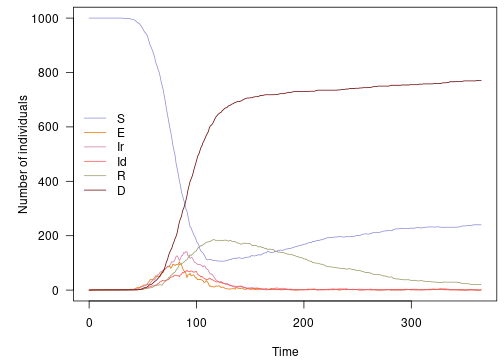Several runs can be obtained without rewriting the model, for instance, to get 100 replicates:

x_res <- as.data.frame(replicate(100, x$run(0:365)[, -1])) dim(x_res)  ##  366 600  x_res[1:6, 1:10]  ## S.1 E.1 Id.1 Ir.1 R.1 D.1 S.2 E.2 Id.2 Ir.2 ## 1 1000 1 0 0 0 0 1000 1 0 0 ## 2 1000 1 0 0 0 0 1000 1 0 0 ## 3 1000 0 0 1 0 0 1000 2 0 0 ## 4 1000 0 0 1 0 0 1000 1 1 0 ## 5 1000 0 0 1 0 0 1000 1 1 0 ## 6 1000 0 0 1 0 0 1000 0 2 0  seirds_col_transp <- paste0(seirds_col, "1A") par(mar = c(4.1, 5.1, 0.5, 0.5), las = 1) matplot(0:365, x_res, xlab = "Time", ylab = "Number of individuals", type = "l", col = rep(seirds_col_transp, 100), lty = 1) legend("right", lwd = 1, col = seirds_col, legend = c("S", "E", "Ir", "Id", "R", "D"), bty = "n")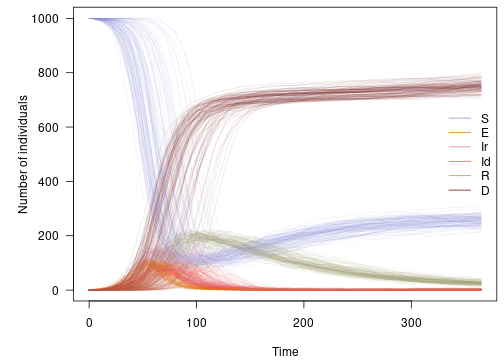It is then possible to explore the behaviour of the model using a simple function: check_model <- function(n = 50, t = 0:365, alpha = 0.2, ..., legend_pos = "topright") { model <- seirds_generator(...) col <- paste0(seirds_col, "33") res <- as.data.frame(replicate(n, model$run(t)[, -1]))
opar <- par(no.readonly = TRUE)
on.exit(par(opar))
par(mar = c(4.1, 5.1, 0.5, 0.5), las = 1)
matplot(t, res, xlab = "Time", ylab = "",  type = "l",
col = rep(col, n), lty = 1)
mtext("Number of individuals", side = 2, line = 3.5, las = 3, cex = 1.2)
legend(legend_pos, lwd = 1, col = seirds_col,
legend = c("S", "E", "Ir", "Id", "R", "D"), bty = "n")
}


This is a sanity check with a null infection rate and no imported case:

check_model(beta = 0, epsilon = 0)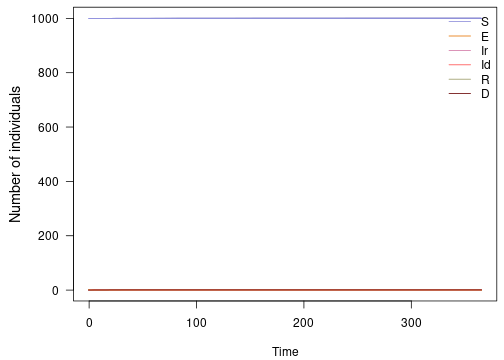Another easy case: no importation, no waning immunity:

check_model(epsilon = 0, omega = 0)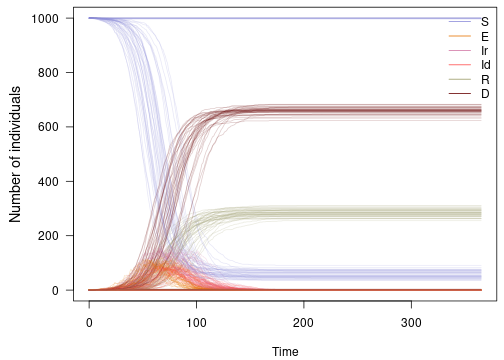A more nuanced case: persistence of the disease with limited import, waning immunity, low severity, larger population:

check_model(t = 0:(365 * 3), epsilon = 0.1, beta = .2, omega = .01,
mu = 0.005, S_ini = 1e5)